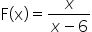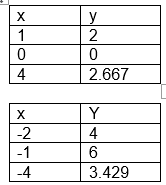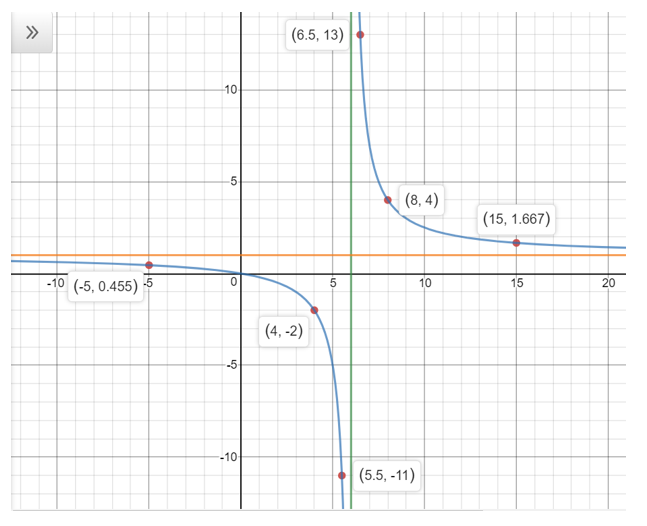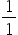Maths-
General
Easy

Question

# Use long Division to rewrite each rational function. Find the asymptotes of  and sketch the graph.Hint:

## The correct answer is: From the graph we can analyze that the vertical asymptote of the rational function is x= 6 and horizontal asymptote is y = (leading coefficient of numerator) / (leading coefficient of denominator) = 1/1=1

### 1.Find the asymptotes of the rational function, if any.2.Draw the asymptotes as dotted lines.3.Find the x -intercept (s) and y -intercept of the rational function, if any.4.Find the values of y for several different values of x .5.Plot the points and draw a smooth curve to connect the points. Make sure that the graph does not cross the vertical asymptotes.The vertical asymptote of a rational function is x -value where the denominator of the function is zero. Equate the denominator to zero and find the value of x .x - 6= 0x = 6The vertical asymptote of the rational function is x= 6We will find more points on the function and graph the function.From the graph we can analyze that the vertical asymptote of the rational function is  x= 6 and horizontal asymptote isy = (leading coefficient of numerator) / (leading coefficient of denominator) ==1#### With Turito Foundation.#### Get an Expert Advice From Turito.